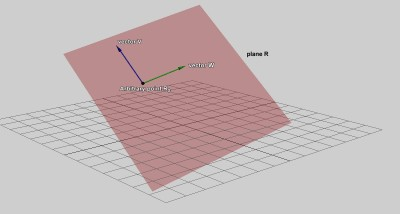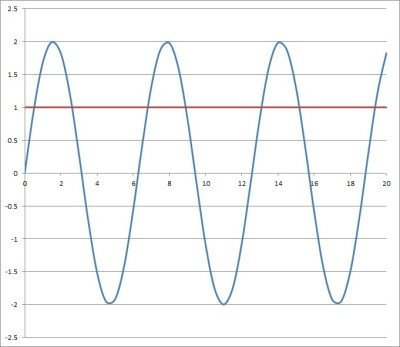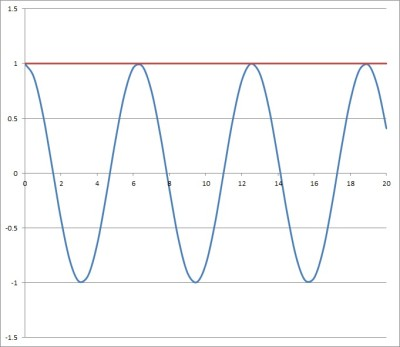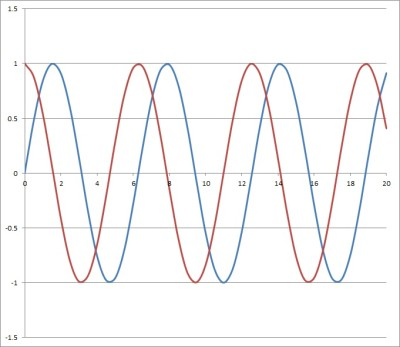# Equations With Infinite Solutions (6 Examples & Explanations)

When solving an equation, we might find that there is no solution, one solution, multiple solutions, or infinite solutions (we can also say “infinitely many solutions”).   It helps to know what some of these look like so you can recognize them in case you come across them.

So, what are some equations with infinite solutions?  Some equations with trig functions (like sin(x) = 0) have infinitely many solutions. There are some equations in one variable (like (x+1)2 = x2+ 2x + 1) that have infinitely many solutions. There are also equations with two or more variables (like x = y) which have infinitely many solutions.

Of course, there are lots of equations with infinite solutions – the few mentioned above are just a few examples.

In this article, we’ll talk about what it means for an equation to have infinite solutions.  We’ll also look at some examples and explain why there are infinite solutions in those cases.

Let’s get started.

## Equations With Infinite Solutions

There are some general indications that an equation may have infinite solutions.  For example:

• If both sides of the equation are the same (or equivalent after rearranging terms), then there are always infinite solutions.  This can occur for equations with one or more variables. For example, 2(x + 3) = 2x + 6 is the same on both sides after we use the Distributive Property on the left.  So, it has infinite solutions.
• If there are two or more variables in the equation, then there may be infinite solutions.  For example, y = x2 has infinite solutions: for any real number x we choose, we can easily find a solution y by squaring x.
• If there is an oscillating or periodic function (such as sine or cosine), then there may be infinitely many solutions.  For example, sin(x) = 0 has infinitely many solutions, since every integer multiple of π radians is a solution (so π, 2π, 3π, 4π, … are all solutions).

*Note: when we say that an equation has infinite solutions (or infinitely many solutions), we do not mean that ∞ is a solution to the equation.  We mean that there exist an unlimited number of solutions to the equation (where each solution is a finite number).

Now let’s look at some examples of equations with infinite solutions, along with an explanation for each one.

### Example 1: An Equation In One Variable With Infinitely Many Solutions

Consider the following equation in one variable:

• (x + 1)2 + 4x = (x + 3)2 – 8

We will need to do some work (using FOIL and combining like terms) to see if there are infinite solutions:

• (x2 + 2x + 1) + 4x = (x + 3)2 – 8  [(x + 1)2 = (x2 + 2x + 1), by FOIL]
• (x2 + 2x + 1) + 4x = (x2 + 6x + 9) – 8  [(x + 3)2 = (x2 + 6x + 9), by FOIL]
• x2 + 6x + 1 = x2 + 6x + 1  [combine like terms on both sides]
• 0 = 0

This last statement is always true, no matter what value of x we choose.  So, there are infinite solutions for the original equation – any real value of x will work!

### Example 2: An Equation In Two Variables With Infinitely Many Solutions

Consider the following equation in two variables:

• y = 2x2 – 5x + 1

There are infinitely many solutions to this equation.  In this case, we can choose any real value for x, and find y by plugging in our chosen value of x into the equation.

For example:

• For x = 0, we get y = 2(0)2 – 5(0) + 1 = 2*0 – 0 + 1 = 0 – 0 + 1 = 1
• For x = 1, we get y = 2(1)2 – 5(1) + 1 = 2*1 – 5 + 1 = 2 – 5 + 1 = -2
• For x = 2, we get y = 2(2)2 – 5(2) + 1 = 2*4 – 10 + 1 = 8 – 10 + 1 = -1
• Etc.

The graph below shows the set of solutions (a parabola, which is the graph of a quadratic).

### Example 3: An Equation In Three Variables With Infinitely Many Solutions

Consider the following equation in two variables:

• z = x + y

There are infinitely many solutions to this equation.  In this case, we can choose any real value for x and any real value for y, and find z by plugging in our chosen values of x and y into the equation.

For example:

• For x = 0 and y = 0, we get z = 0 + 0 = 0
• For x = 0 and y = 1, we get z = 0 + 1 = 1
• For x = 1 and y = 1, we get z = 1 + 1 = 2
• Etc.

The graph of the equation z = x + y would be an entire plane when graphed in 3D space.A plane (such as z = x + y) is graphed in 3D space. There are infinite solutions to the equation z = x + y.

### Example 4: An Equation With Trig Functions With Infinitely Many Solutions

Consider the following equation with a trigonometric function:

• 2sin(x) = 1
• sin(x) = ½
• x = (12k + 1)π/6, (12k + 5)π/6 for any integer k

Since k can be any integer, there are infinitely many solutions for the equation.  You can see the graph showing some of the points where y = 2sin(x) and y = 1 intersect below.This shows some of the solutions for the equation 2sin(x) = 1. The blue curve is part of the graph of y = 2sin(x), and the red line is the horizontal line y = 1. There are infinite solutions to the equation 2sin(x) = 1.

Note that the same type of pattern will hold for any periodic function (sine, cosine, etc.)

### Example 5: An Equation With Trig Functions With Infinitely Many Solutions

Consider the following equation with a trigonometric function:

• cos(x) = 1
• x = kπ for any integer k

Since k can be any integer, there are infinitely many solutions for the equation.  You can see the graph showing some of the points where y = cos(x) and y = 1 intersect below.This shows some of the solutions for the equation cos(x) = 1. The blue curve is part of the graph of y = cos(x), and the red line is the horizontal line y = 1. There are infinite solutions to the equation cos(x) = 1.

### Example 6: An Equation With Trig Functions With Infinitely Many Solutions

Consider the following equation with a trigonometric function:

• sin(x) = cos(x)
• sin(x)/cos(x) = cos(x)/cos(x)
• tan(x) = 1

This occurs when x = (8k+1)π/4 and x = (8k+5)π/4 for every integer k.

*Note: since we divided by cos(x), we should check the case when cos(x) = 0, which occurs when x = kπ/2 for every k.  In that case, sin(x) is 1, which is not equal to 0.

Since k can be any integer, there are infinitely many solutions for the equation.  You can see the graph showing some of the points where y = cos(x) and y = 1 intersect below.This shows some of the solutions for the equation sin(x) = cos(x). The blue curve is part of the graph of y = sin(x), and the red line is part of the graph of y = cos(x). There are infinite solutions to the equation sin(x) = cos(x).

## Conclusion

Now you know about some equations that have infinite solutions and what they look like.